# Difference between revisions of "Credit Value at Risk"

## Definition

Credit Value at Risk (CVaR) is a Risk Measure that aims to capture the downside value risk of a Credit Portfolio.

CVaR is a quantile measure and requires the specification of

• An aggregate Portfolio Loss (or Profit and Loss) variable constructed as the sum of potential individual losses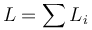$L=\sum L_{i}$
• A Confidence Level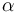$\alpha$

Given a confidence level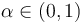$\alpha\in(0,1)$, the CVaR of calculated portfolio loss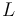$L$ at the confidence level$\alpha$ is the smallest number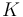$K$ such that the probability that the loss$L$ exceeds$K$ is at least$\alpha$.

## Usage

Credit Value at Risk is used in conjunction with a Credit Portfolio Model, a computational approach for the generation of future scenarios including different risk realizations.

## Issues and Challenges

• CVaR has been developed largely as a mirror of the Value at Risk measure for portfolios of marketable securities, yet the more illiquid nature of credit portfolios forces substantial further assumptions and associated Model Risk
• CVaR inherits the weaknesses of VaR frameworks